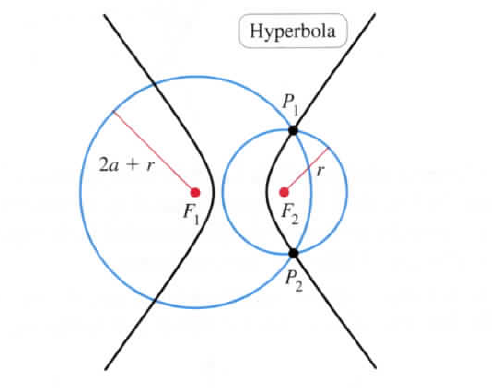×
Get Full Access to Calculus: Early Transcendentals - 1 Edition - Chapter 10.4 - Problem 75e
Get Full Access to Calculus: Early Transcendentals - 1 Edition - Chapter 10.4 - Problem 75e

×

# Another construction for a hyperbola Suppose two circlesISBN: 9780321570567 2

## Solution for problem 75E Chapter 10.4

Calculus: Early Transcendentals | 1st Edition

• Textbook Solutions
• 2901 Step-by-step solutions solved by professors and subject experts
• Get 24/7 help from StudySoup virtual teaching assistantsCalculus: Early Transcendentals | 1st Edition

4 5 1 396 Reviews
26
3
Problem 75E

Suppose two circles are centered at $$F_1$$ and $$F_2$$, respectively, whose centers are at least 2a units apart (see figure). The radius of one circle is 2a + r and the radius of the other circle is r, where $$r\ \geq\ 0$$. Show that as r increases, the intersection point P of the two circles describes one branch of a hyperbola with foci at $$F_1$$ and $$F_2$$.Step-by-Step Solution:

Solution 75E

Step 1:

Given that

Suppose two circles are centered at F1 and F2, respectively, whose centers are at least 2a units apart (see figure). The radius of one circle is 2a + r and the radius of the other circle is r, where r ≥ 0.Step 2 of 7

Step 3 of 7

##### ISBN: 9780321570567

The full step-by-step solution to problem: 75E from chapter: 10.4 was answered by , our top Calculus solution expert on 03/03/17, 03:45PM. This full solution covers the following key subjects: circle, radius, hyperbola, Circles, increases. This expansive textbook survival guide covers 112 chapters, and 5248 solutions. The answer to “Another construction for a hyperbola Suppose two circles are centered at F1 and F2, respectively, whose centers are at least 2a units apart (see figure). The radius of one circle is 2a + r and the radius of the other circle is r, where r ? 0. Show that as r increases, the intersection point P of the two circles describes one branch of a hyperbola with Foci at F1 and F2.” is broken down into a number of easy to follow steps, and 72 words. Calculus: Early Transcendentals was written by and is associated to the ISBN: 9780321570567. Since the solution to 75E from 10.4 chapter was answered, more than 361 students have viewed the full step-by-step answer. This textbook survival guide was created for the textbook: Calculus: Early Transcendentals, edition: 1.

Unlock Textbook Solution